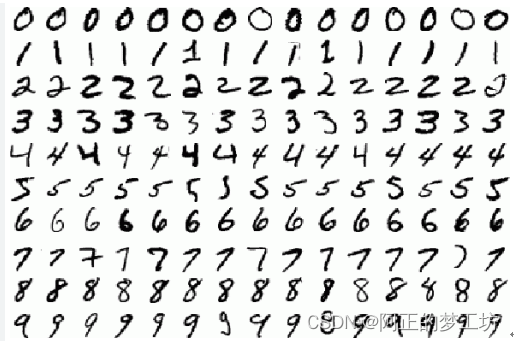##MSIPO技术圈 首页 IT技术 查看内容

# PyTorch训练RNN, GRU, LSTM：手写数字识别

2023-07-13

## pytorch 神经网络训练demo``````# Imports
import torch
import torch.nn as nn
import torch.optim as optim
import torch.nn.functional as F
import torchvision.datasets as datasets
import torchvision.transforms as transforms

# Set device
device = torch.device('cuda' if torch.cuda.is_available() else 'cpu')

# Hyperparameters
input_size = 28
sequence_length = 28
num_layers = 2
hidden_size = 256
num_classes = 10
learning_rate = 0.001
batch_size = 64
num_epochs = 2

# Create a RNN
class RNN(nn.Module):
def __init__(self, input_size, hidden_size, num_layers, num_classes):
super(RNN, self).__init__()
self.hidden_size = hidden_size
self.num_layers = num_layers
self.rnn = nn.RNN(input_size, hidden_size, num_layers, batch_first=True)
self.fc = nn.Linear(hidden_size*sequence_length, num_classes) # fully connected

def forward(self, x):
h0 = torch.zeros(self.num_layers, x.size(0), self.hidden_size).to(device)

# Forward Prop
out, _ = self.rnn(x, h0)
out = out.reshape(out.shape, -1)
out = self.fc(out)

return out

# Create a GRU
class RNN_GRU(nn.Module):
def __init__(self, input_size, hidden_size, num_layers, num_classes):
super(RNN_GRU, self).__init__()
self.hidden_size = hidden_size
self.num_layers = num_layers
self.gru = nn.GRU(input_size, hidden_size, num_layers, batch_first=True)
self.fc = nn.Linear(hidden_size*sequence_length, num_classes) # fully connected

def forward(self, x):
h0 = torch.zeros(self.num_layers, x.size(0), self.hidden_size).to(device)

# Forward Prop
out, _ = self.gru(x, h0)
out = out.reshape(out.shape, -1)
out = self.fc(out)

return out

# Create a LSTM
class RNN_LSTM(nn.Module):
def __init__(self, input_size, hidden_size, num_layers, num_classes):
super(RNN_LSTM, self).__init__()
self.hidden_size = hidden_size
self.num_layers = num_layers
self.lstm = nn.LSTM(input_size, hidden_size, num_layers, batch_first=True)
self.fc = nn.Linear(hidden_size*sequence_length, num_classes) # fully connected

def forward(self, x):
h0 = torch.zeros(self.num_layers, x.size(0), self.hidden_size).to(device)
c0 = torch.zeros(self.num_layers, x.size(0), self.hidden_size).to(device)

# Forward Prop
out, _ = self.lstm(x, (h0, c0))
out = out.reshape(out.shape, -1)
out = self.fc(out)
return out

train_dataset = datasets.MNIST(root='dataset/',
train=True,
transform=transforms.ToTensor(),
test_dataset = datasets.MNIST(root='dataset/',
train=False,
transform=transforms.ToTensor(),

# Initialize network 选择一个即可
model = RNN(input_size, hidden_size, num_layers, num_classes).to(device)
# model = RNN_GRU(input_size, hidden_size, num_layers, num_classes).to(device)
# model = RNN_LSTM(input_size, hidden_size, num_layers, num_classes).to(device)

# Loss and optimizer
criterion = nn.CrossEntropyLoss()

# Train network
for epoch in range(num_epochs):
# data: images, targets: labels
for batch_idx, (data, targets) in enumerate(train_loader):
# Get data to cuda if possible
data = data.to(device).squeeze(1) # 删除一个张量中所有维数为1的维度 (N, 1, 28, 28) -> (N, 28, 28)
targets = targets.to(device)

# forward
scores = model(data) # 64*10
loss = criterion(scores, targets)

# backward
loss.backward()

optimizer.step()

# Check accuracy on training & test to see how good our model
print("Checking accuracy on training data")
else:
print("Checking accuracy on test data")
num_correct = 0
num_samples = 0
model.eval()

x = x.to(device).squeeze(1)
y = y.to(device)
# x = x.reshape(x.shape, -1) # 64*784

scores = model(x)# 64*10
_, predictions = scores.max(dim=1) #dim=1，表示对每行取最大值，每行代表一个样本。
num_correct += (predictions == y).sum()
num_samples += predictions.size(0) # 64

print(f'Got {num_correct} / {num_samples} with accuracy {float(num_correct)/float(num_samples)*100:.2f}%')

model.train()

``````

## Result

``````RNN Result
Checking accuracy on training data
Got 57926 / 60000 with accuracy 96.54%
Checking accuracy on test data
Got 9640 / 10000 with accuracy 96.40%

GRU Result
Checking accuracy on training data
Got 59058 / 60000 with accuracy 98.43%
Checking accuracy on test data
Got 9841 / 10000 with accuracy 98.41%

LSTM Result
Checking accuracy on training data
Got 59248 / 60000 with accuracy 98.75%
Checking accuracy on test data
Got 9849 / 10000 with accuracy 98.49%
``````